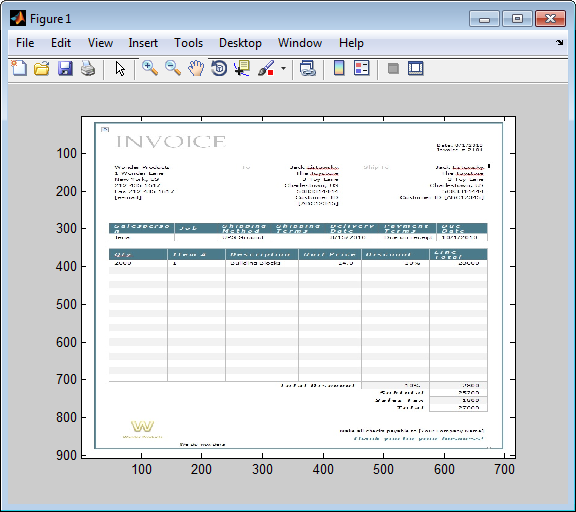Main Content

## Retrieve Image Data Types

This example shows how to retrieve images from a Microsoft® Access™ database. To run this example, define the function `parsebinary` using this code.

```function [x,map] = parsebinary(o,f) %PARSEBINARY Write binary object to disk and display if image. % [X,MAP] = PARSEBINARY(O,F) writes the binary object in O to disk % in the format specified by F. If the object is an image, % display the image. This file was released for demonstration % purposes only. A Microsoft(R) Access(TM) database contains image data. % Use an ODBC driver to read the data. This function writes % any temporary files to the current working directory. % % Valid file formats are: % % BMP Bitmap % DOC Microsoft(R) Word document % GIF GIF file % PPT Microsoft(R) Powerpoint(R) file % TIF TIF file % XLS Microsoft(R) Excel(R) spreadsheet % PNG Portable Network Graphics % Transform object into vector of data v = java.util.Vector; v.addElement(o); bdata = v.elementAt(0); % Open file to write data to disk fid = fopen(['testfile.' lower(f)],'wb'); % n specifies the end point of data written to disk n = length(bdata); % File type determines how many bytes of header data that % the ODBC driver prepended to the data. switch lower(f) case 'bmp' m = 79; case 'doc' m = 86; case 'gif' m = 5722; case 'png' m = 182; n = length(bdata)-285; case 'ppt' m = 94; case 'tif' m = 6472; case 'xls' m = 83; otherwise error(message('database:parsebinary:unknownFormat')) end % Write data to disk fwrite(fid,bdata(m:n),'int8'); fclose(fid); % Display if image switch lower(f) case {'bmp','tif','gif','png'} [x,map] = imread(['testfile.' lower(f)]); imagesc(x) colormap(map) case {'doc','xls','ppt'} % Microsoft(R) Office formats % Insert path to Microsoft(R) Word or Microsoft(R) Excel(R) % executable here to run from MATLAB(R) prompt. % For example: % !d:\msoffice\winword testfile.doc end```
1. Connect to the Microsoft Access data source using the ODBC driver. The database contains the table `Invoice`.

```conn = database('datasource','',''); ```
2. Import the `InvoiceNumber` and `Receipt` columns of data from `Invoice`.

```sqlquery = 'SELECT InvoiceNumber,Receipt FROM Invoice'; results = fetch(conn,sqlquery); ```
3. View the imported data.

`results`
```ans = 10×2 table InvoiceNumber Receipt _____________ _________________ 2101 [1948410×1 uint8] 3546 [2059994×1 uint8] 33116 [ 487034×1 uint8] 34155 [2059994×1 uint8] 34267 [2454554×1 uint8] 37197 [1926362×1 uint8] 37281 [2403674×1 uint8] 41011 [1920474×1 uint8] 61178 [2378330×1 uint8] 62145 [ 492314×1 uint8] ```
4. Assign the image element you want to the variable `receipt`.

```receipt = results.Receipt{1}; ```
5. Run the `parsebinary` function. The function writes retrieved data to a file, strips ODBC header information from it, and displays `receipt` as a bitmap image in a figure window. Ensure that your current folder is writable so that the `parsebinary` function can write the output data.

```cd 'I:\MATLABFiles\myfiles' parsebinary(receipt,'BMP'); ```## Support

#### Using MATLAB with Big Data from Sensors and IoT Devices

Download white paper